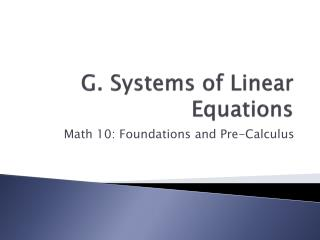DownloadDownload PresentationG. Systems of Linear Equations

# G. Systems of Linear Equations

Download Presentation## G. Systems of Linear Equations

- - - - - - - - - - - - - - - - - - - - - - - - - - - E N D - - - - - - - - - - - - - - - - - - - - - - - - - - -
##### Presentation Transcript

1. G. Systems of Linear Equations Math 10: Foundations and Pre-Calculus

2. Key Terms: • Find the definition of the following terms: • Systems of Linear Equations • Linear System • Solving by Substitution • Equivalent Systems • Solving by Elimination • Infinite • Coincident Lines

3. 1. Developing a System of Linear Equations

4. A school district has buses that carry 12 students and buses that carry 24 students. The total capacity is 780. There are 20 more small buses than large buses. How many of each are there?

5. To determine how many of each bus there is we can write two equations to model the situation. • First what are the unknown quantities. • Now lets use this info to set up an equation.

6. Now lets use the other info to find the second equation.

7. These 2 equations form a system of linear equations in 2 variables, s and l. Also referred to as a linear system. • A solution of a linear system is a pair of values of s and lthat satisfy both equations

8. Suppose you were told there were 35 small buses and 15 large buses. To verify first see if it makes sense in the given data. • Our answers agree with the given data, so our selection is correct. • We can also verify our solutions by subbing the known values of s and l into the equations we made

9. For each equation, the left side is the same as the right side so our answers are correct.

10. Example

11. Example

12. The example situation on p. 399 shows that it is better to consider the given data to verify a solution first then check using the equations.

13. Example

14. Practice • Ex. 7.1 (p. 400) #1-14 #4-18

15. 2. Solving Systems of Equations Graphically

16. The solution of a linear system can be estimated by using graphing. • If the 2 lines intersect, the coordinates (x,y) of the point of intersection are the solution to the system

17. Lets graph the following system to find the solution:

18. The point of intersection appears to be (-2,-3) • To verify the solution, check to see if the coordinate satisfies the equation

19. Example

20. Example

21. Example

22. Practice • Ex. 7.2 (p. 408) #1-16 odds in each #4-19 odds in each

23. 3. The World of Technology • Construct Understanding p. 411

24. Practice • Ex. 7.3 (p. 412) #1-5 odds in each

25. 4. Using Substitution to Solve

26. Using graphing to solve systems of equations is time consuming and we most times are only getting an approximate answer • We can use algebra to get an exact solution and one algebraic strategy is called solving by substitution

27. By using substitution, we transform a system of 2 linear equations into a single equation in one variable. • Then we use what we know about solving linear equations to determine the value of the variable

28. Consider this system:

29. Verify by substituting into original equation.

30. Example

31. Example

32. They have the same solution because they are equivalent equations in each system • Multiplying or dividing the equations in a linear system by a non-zero number does not change the graphs. • So their point of intersection, and hence, their solution is unchanged.

33. A system of equivalent equations is called an equivalent linear system and has the same solution as the original

34. When an equation in a linear system has coefficients or a constant term that are fractions, we can multiply by a common denominator to write an equivalent equation with integer coefficients • Basically we get ride of the fractions

35. Example

36. Practice • Ex. 7.4 (p. 424) #1-5, 7-14, 17-19, 21 #4-23 do the odds in the question that have multiply questions

37. 5. Solving Systems of Equations using Elimination • Construct Understanding p. 429

38. Similarly to the last section that we looked at, adding or subtracting 2 equations in a linear system produces equivalent linear systems. • We use this property to solve a linear system by first eliminating one variable by adding or subtracting the two equations. • This is called Solving by Elimination

39. Consider the systems below:

40. We may need to multiply one or both equations by a constant before we can eliminate a variable by adding or subtracting

41. Example

42. In a linear system, we may have to write equivalent equations with integer coefficients before we apply the elimination strategy

43. Example

44. Example

45. Example